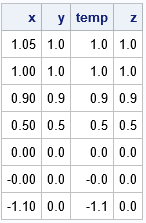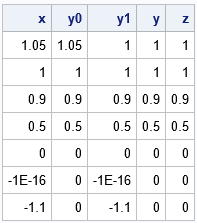Finite-precision computations can be tricky. You might know, mathematically, that a certain result must be non-negative or must be within a certain interval. However, when you actually compute that result on a computer that uses finite-precision, you might observe that the value is slightly negative or slightly outside of the interval. This frequently happens when you are adding numbers that are not exactly representable in base 2. One of the most famous examples is the sum
0.1 + 0.1 + 0.1 ≠ 0.3 (finite precision),
which is shown to every student in Computer Science 101. Other examples include:

• If x is in the interval [0,1], then y = 1 - x10 is also in the interval [0,1]. That is true in exact precision, but not necessarily true in finite-precision computations when x is close to 1.
• Although sin2(t) + cos2(t) = 1 in exact arithmetic for all values of t, the equation might not be true in finite precision.

SAS programs that demonstrate the previous finite-precision computations are shown at the end of this article. Situations like these can cause problems when you want to use the result in a function that has a restricted domain. For example, the SQRT function cannot operate on negative numbers. For probability distributions, the quantile function cannot operate on numbers outside the interval [0,1].

This article discusses how to "trap" results that are invalid because they are outside of a known interval. You can use IF-THEN/ELSE logic to catch an invalid result, but SAS provides more compact syntax. This article discusses using the IFN function in Base SAS and using the "elementwise minimum" (<>) and "elementwise maximum" (><) operators in the SAS/IML language.

### Trap and map

I previously wrote about the "trap and cap" technique for handling functions like log(x). The idea is to "trap" invalid input values (x ≤ 0) and "cap" the output when you are trying to visualize the function. For the present article, the technique is "trap and map": you need to detect invalid computations and then map the result back into the interval that you know is mathematically correct. For example, if the argument is supposed to represent a probability in the interval [0,1], you must map negative numbers to 0 and map numbers greater than 1 to 1.

### How to use the IFN function in SAS

Clearly, you can "trap and map" by using IF-THEN/ELSE logic. For example, suppose you know that a variable, x, must be in the interval [0,1]. You can use the SAS DATA step to trap invalid values and map them into the interval, as follows:

```/* trap invalid values of x and map them into [0,1]. Store result in y */ data Map2; input x @@; if x<0 then y = 0; else if x>1 then y = 1; else y = x; datalines; 1.05 1 0.9 0.5 0 -1e-16 -1.1 ;```

This program traps the invalid values, but it requires six lines of IF-THEN/ELSE logic. A more compact syntax is to use the IFN function. The IFN function has three arguments:

• The first argument is a logical expression, such as x < 0.
• The second argument is the value to return when the logical expression is true.
• The third argument is the value to return when the logical expression is false.

For example, you can use the function call IFN(x<0, 0, x) to trap negative values of x and map those values to 0. Similarly, you can use the function call IFN(x>1, 1, x) to trap values of x that are greater than 1 and map those values to 1. To perform both of the trap-and-map operations on one line, you can nest the function calls so that the third argument to the IFN function is itself a function call, as follows:

```data Map2; input x @@; y = ifn(x<0, 0, ifn(x>1, 1, x)); /* trap and map: force x into [0,1] */   /* or, for clarity, split into two simpler calls */ temp = ifn(x>1, 1, x); /* force x <= 1 */ z = ifn(x<0, 0, temp); /* force x >= 0 */ datalines; 1.05 1 0.9 0.5 0 -1e-16 -1.1 ;   proc print noobs; run;```The output shows that the program performed the trap-and-map operation correctly. All values of y are in the interval [0,1].

If you can't quite see how the logic works in the statement that nests the two IFN calls, you can split the logic into two steps as shown later in the program. The first IFN call traps any variables that are greater than 1 and maps them to 1. The second IFN call traps any variables that are less than 0 and maps them to 0. The z variable has the same values as the y variable.

### How to use the element min/max operators in SAS/IML

The SAS/IML language contains operators that perform similar computations. If x is a vector of numbers, then

• The expression (x <> 0) returns a vector that is the elementwise maximum between the elements of x and 0. In other words, any elements of x that are less than 0 get mapped to 0.
• The expression (x >< 1) returns a vector that is the elementwise minimum between the elements of x and 1. In other words, any elements of x that are greater than 1 get mapped to 1.
• You can combine the operators. The expression (x <> 0) >< 1 forces all elements of x into the interval [0,1]. Because the elementwise operators are commutative, you can also write the expression as 0 <> x >< 1.

These examples are shown in the following SAS/IML program:

```proc iml; x = {1.05, 1, 0.9, 0.5, 0, -1e-16, -1.1}; y0 = (x <> 0); /* force x >= 0 */ y1 = (x >< 1); /* force x <= 1 */ y01 = (x <> 0) >< 1; /* force x into [0,1] */ print x y0 y1 y01;```### Summary

In summary, because of finite-precision computations (or just bad input data), it is sometimes necessary to trap invalid values and map them into an interval. Using IF-THEN/ELSE statements is clear and straightforward, but require multiple lines of code. You can perform the same logical trap-and-map calculations more compactly by using the IFN function in Base SAS or by using the elementwise minimum or maximum operators in SAS/IML.

### Appendix: Examples of floating-point computations to study

The following SAS DATA step programs show typical computations for which the floating-point computation can produce results that are different from the expected mathematical (full-precision) computation.

```data FinitePrecision; z = 0.1 + 0.1 + 0.1; if z^=0.3 then put 'In finite precision: 0.1 + 0.1 + 0.1 ^= 0.3'; run;   data DomainError1; do t = -4 to 4 by 0.1; x = cos(t); y = sin(t); z = x**2 + y**2; /* always = 1, mathematically */ s = sqrt(1 - z); /* s = sqrt(0) */ output; end; run;   data DomainError2; do x = 0 to 1 by 0.01; z = 1 - x**10; /* always in [0,1], mathematically */ s = sqrt(z); output; end; run;```